# Title 40

## SECTION 1065.642

### 1065.642 PDP, SSV, and CFV molar flow rate calculations.

§ 1065.642 PDP, SSV, and CFV molar flow rate calculations.

This section describes the equations for calculating molar flow rates from various flow meters. After you calibrate a flow meter according to § 1065.640, use the calculations described in this section to calculate flow during an emission test.

(a) PDP molar flow rate. (1) Based on the speed at which you operate the PDP for a test interval, select the corresponding slope, a1, and intercept, a0, as calculated in § 1065.640, to calculate PDP molar flow rate,, as follows: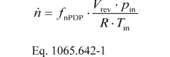Where: fnPDP = pump speed. Vrev = PDP volume pumped per revolution, as determined in paragraph (a)(2) of this section. pin = static absolute pressure at the PDP inlet. R = molar gas constant. Tin = absolute temperature at the PDP inlet.

(2) Calculate Vrev using the following equation: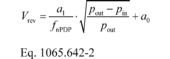pout = static absolute pressure at the PDP outlet. Example: a1 = 0.8405 (m 3/s) fnPDP = 12.58 r/s Pout = 99.950 kPa Pin = 98.575 kPa = 98575 Pa = 98575 kg/(m·s 2) a0 = 0.056 (m 3/r) R = 8.314472 J/(mol·K) = 8.314472 (m 2·kg)/(s 2·mol·K) Tin = 323.5 K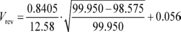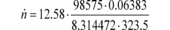n = 29.428 mol/s

(b) SSV molar flow rate. Calculate SSV molar flow rate, n , as follows: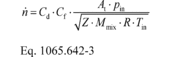Where: Cd = discharge coefficient, as determined based on the Cd versus Re# equation in § 1065.640(d)(2). Cf = flow coefficient, as determined in § 1065.640(c)(2)(ii). At = venturi throat cross-sectional area. Pin = static absolute pressure at the venturi inlet. Z = compressibility factor. Mmix = molar mass of gas mixture. R = molar gas constant. Tin = absolute temperature at the venturi inlet. Example: At = 0.01824 m 2 pin = 99.132 kPa = 99132 Pa = 99132 kg/(m·s 2) Z = 1 Mmix = 28.7805 g/mol = 0.0287805 kg/mol R = 8.314472 J/(mol·K) = 8.314472 (m 2·kg)/(s 2·mol·K) Tin = 298.15 K Re# = 7.232·10 5 g = 1.399 b = 0.8 Δp = 2.312 kPa

Using Eq. 1065.640-7, rssv = 0.997

Using Eq. 1065.640-6, Cf = 0.274

Using Eq. 1065.640-5, Cd = 0.990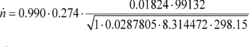n = 58.173 mol/s

(c) CFV molar flow rate. If you use multiple venturis and you calibrate each venturi independently to determine a separate discharge coefficient, Cd (or calibration coefficient, Kv), for each venturi, calculate the individual molar flow rates through each venturi and sum all their flow rates to determine CFV flow rate, n . If you use multiple venturis and you calibrated venturis in combination, calculate n using the sum of the active venturi throat areas as At, the square root of the sum of the squares of the active venturi throat diameters as dt, and the ratio of the venturi throat to inlet diameters as the ratio of the square root of the sum of the active venturi throat diameters (dt) to the diameter of the common entrance to all the venturis (D).

(1) To calculate n through one venturi or one combination of venturis, use its respective mean Cd and other constants you determined according to § 1065.640 and calculate n as follows: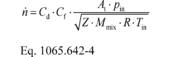Example: Cd = 0.985 Cf = 0.7219 At = 0.00456 m 2 pin = 98.836 kPa = 98836 Pa = 98836 kg/(m·s 2) Z = 1 Mmix = 28.7805 g/mol = 0.0287805 kg/mol R = 8.314472 J/(mol·K) = 8.314472 (m 2·kg)/(s 2·mol·K) Tin = 378.15 K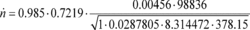n = 33.690 mol/s

(2) To calculate the molar flow rate through one venturi or a combination of venturis, you may use its respective mean, Kv, and other constants you determined according to § 1065.640 and calculate its molar flow rate n during an emission test. Note that if you follow the permissible ranges of dilution air dewpoint versus calibration air dewpoint in Table 3 of § 1065.640, you may set Mmix-cal and Mmix equal to 1. Calculate n as follows: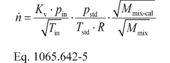Where: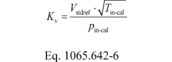Vstdref = volume flow rate of the standard at reference conditions of 293.15 K and 101.325 kPa. Tin-cal = venturi inlet temperature during calibration. Pin-cal = venturi inlet pressure during calibration. Mmix-cal = molar mass of gas mixture used during calibration. Mmix = molar mass of gas mixture during the emission test calculated using Eq. 1065.640-9. Example: Vstdref = 0.4895 m 3 Tin-cal = 302.52 K Pin-cal = 99.654 kPa = 99654 Pa = 99654 kg/(m·s 2) pin = 98.836 kPa = 98836 Pa = 98836 kg/(m·s 2) pstd = 101.325 kPa = 101325 Pa = 101325 kg/(m·s 2) Mmix-cal = 28.9656 g/mol = 0.0289656 kg/mol Mmix = 28.7805 g/mol = 0.0287805 kg/mol Tin = 353.15 K Tstd = 293.15 K R = 8.314472 J/(mol·K) = 8.314472 (m 2·kg)/(s 2·mol·K)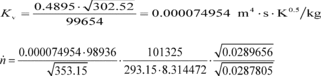n = 16.457 mol/s [81 FR 74177, Oct. 25, 2016]